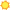# Related Occupations for Task15-2041.01 - Biostatisticians15-2031.00 Operations Research AnalystsBright Outlook   2 closely related tasks Define data requirements and gather and validate information, applying judgment and statistical tests. Break systems into their components, assign numerical values to each component, and examine the mathematical relationships between them. 15-2021.00 MathematiciansApply mathematical theories and techniques to the solution of practical problems in business, engineering, the sciences, or other fields. 15-2041.00 StatisticiansAdapt statistical methods to solve specific problems in many fields, such as economics, biology, and engineering. 15-2091.00 Mathematical Technicians Apply standardized mathematical formulas, principles, and methodology to the solution of technological problems involving engineering or physical science. 15-1132.00 Software Developers, ApplicationsDesign, develop and modify software systems, using scientific analysis and mathematical models to predict and measure outcome and consequences of design.